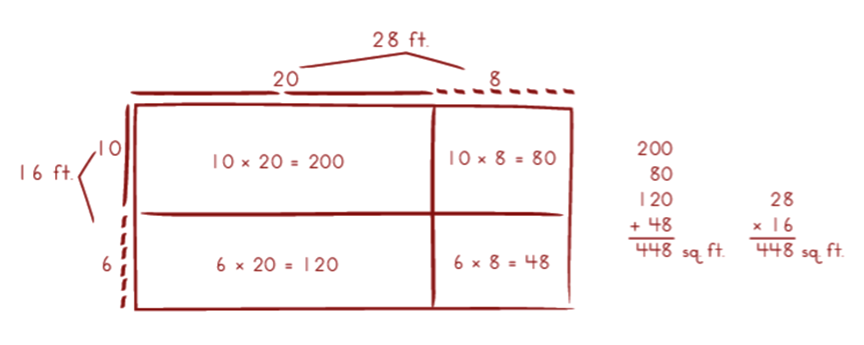# Set 4 Multiplying & Dividing Whole Numbers & DecimalsThis is a strategy I like to use to solve some multiplication problems.

What do you notice about it?This is called the partial products strategy.

I break each factor apart according to place value and then multiply the parts. Then, I add up the partial products to solve the problem.

Let’s try using this strategy!